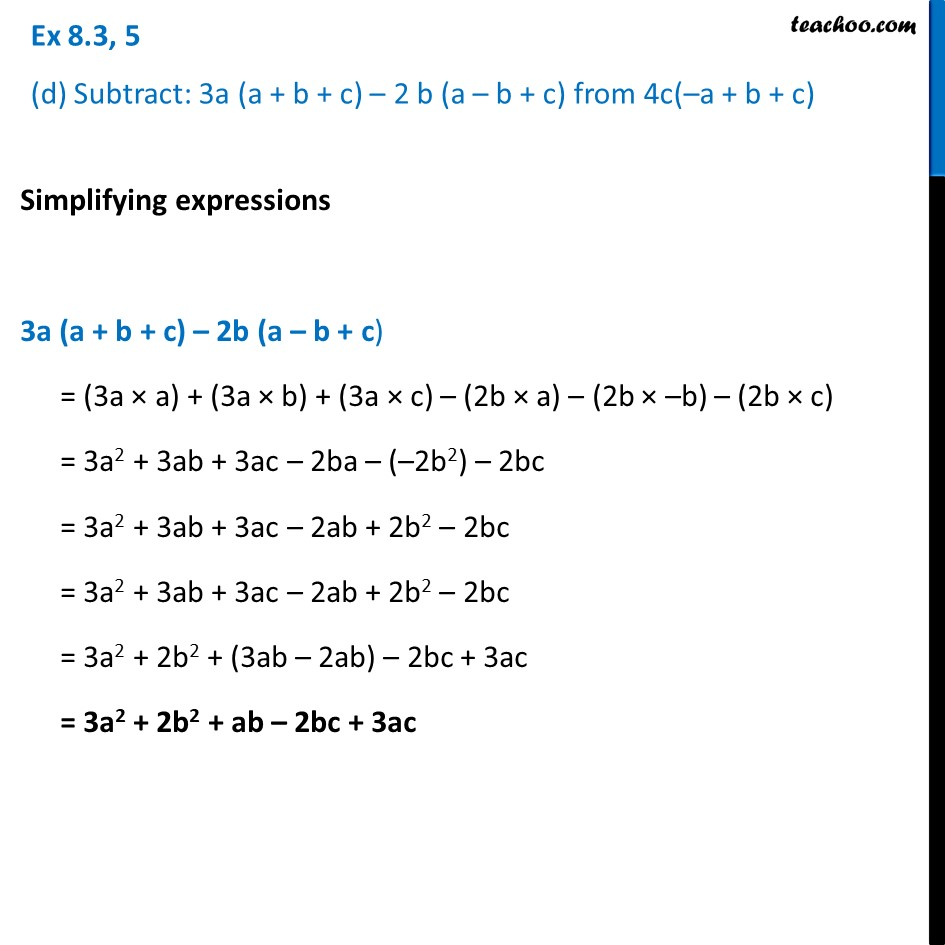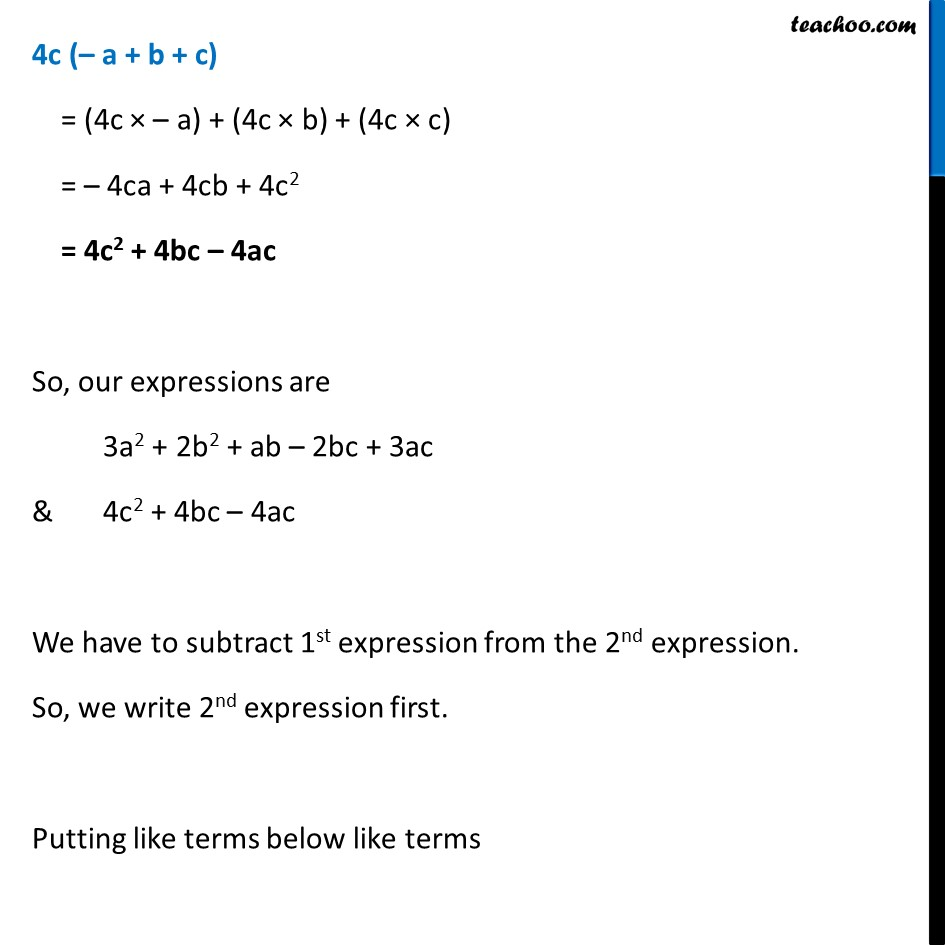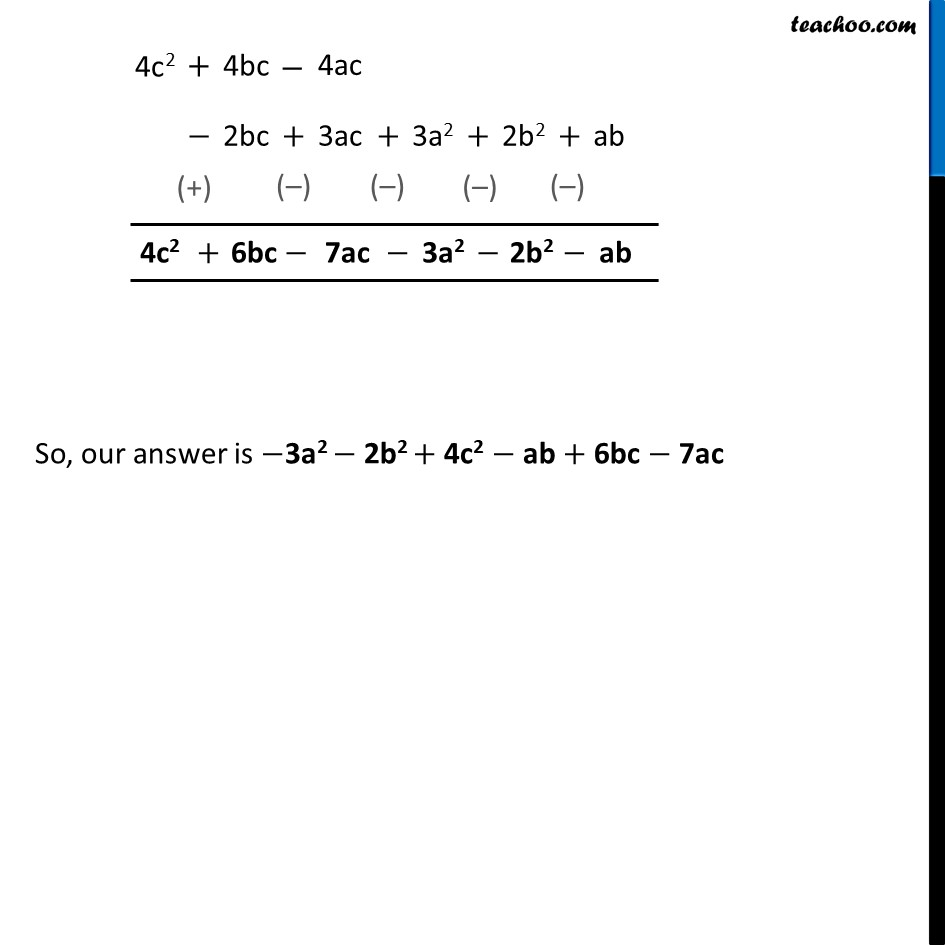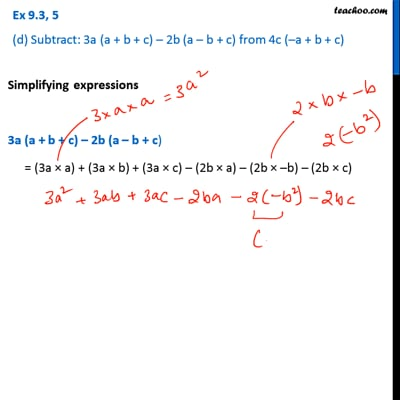Ex 8.3

Chapter 8 Class 8 Algebraic Expressions and Identities
Serial order wiseThis video is only available for Teachoo black users

Learn in your speed, with individual attention - Teachoo Maths 1-on-1 Class

### Transcript

Ex 8.3, 5 (d) Subtract: 3a (a + b + c) – 2 b (a – b + c) from 4c(–a + b + c)Simplifying expressions 3a (a + b + c) – 2b (a – b + c) = (3a × a) + (3a × b) + (3a × c) – (2b × a) – (2b × –b) – (2b × c) = 3a2 + 3ab + 3ac – 2ba – (–2b2) – 2bc = 3a2 + 3ab + 3ac – 2ab + 2b2 – 2bc = 3a2 + 3ab + 3ac – 2ab + 2b2 – 2bc = 3a2 + 2b2 + (3ab – 2ab) – 2bc + 3ac = 3a2 + 2b2 + ab – 2bc + 3ac 4c (– a + b + c) = (4c × – a) + (4c × b) + (4c × c) = – 4ca + 4cb + 4c2 = 4c2 + 4bc – 4ac So, our expressions are 3a2 + 2b2 + ab – 2bc + 3ac & 4c2 + 4bc – 4ac We have to subtract 1st expression from the 2nd expression. So, we write 2nd expression first. Putting like terms below like terms So, our answer is −3a2 − 2b2 + 4c2 − ab + 6bc − 7ac